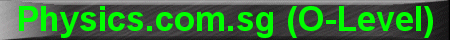(New tips are continually added to these pages.  Check back in a few months' time for more)

TOPIC 7:    Thermal Properties

Tip 1:

Specific Heat Capacity

Let's look at Q = mcDq = CDq.

Q is the amount of thermal energy (measured in joules) required to raise the temperature of a substance of mass m and specific heat capacity c by q degree Celsius or kelvins.

The capital C, equal to mc, is the heat capacity of the entire substance of mass m, which is the amount of thermal energy required to raise the temperature of the entire substance by 1 degree Celsius or kelvin.

An object that has a lower heat capacity C will be more easily heated up turning red hot compared to another object with a higher heat capacity, when given the same amount of heat.

Similarly, a student with lower 'homework capacity' C will be more easily heated up with face turning red hot compared to another student with a higher 'homework capacity', when given the same amount of homework.

Tip 2:

Specific Latent Heat of Fusion/Vaporisation

As regards latent heat of fusion/ vaporisation formula (Q = ml = L), Q is the amount of thermal energy needed to melt/ boil off completely a mass m of a substance (eg. water). So if a substance has a higher latent heat of fusion/ vaporisation, then it will take much more thermal energy (Q) to melt/ boil it, compared to another substance with a lower latent heat of fusion/ vaporisation. (Note that while melting/ boiling, the temperature of the substance remains constant, until it is completely melted/ boiled off).